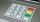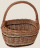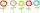# Roman numerals 2-

Subtract up the number written in Roman numerals. Write the results as Roman numbers.

Result

CCCLXIV - LIX = (Correct answer is: CCCV)CLXXXVII - XCIX = (Correct answer is: LXXXVIII)CMLXI - DLXII = (Correct answer is: CCCXCIX)CCCXXVI - XXVI = (Correct answer is: CCC)CCXLIII - CCXXX = (Correct answer is: XIII)CDLXXVI - CXXXI = (Correct answer is: CCCXLV)DCCCVI - DCCLXXI = (Correct answer is: XXXV)CDX - CCCLXX = (Correct answer is: XL)CCCXCVI - CIV = (Correct answer is: CCXCII)CDLXIII - CLXVIII = (Correct answer is: CCXCV)### Step-by-step explanation:

CCCLXIV - LIX = 364 - 59 = 305 = CCCV
CLXXXVII - XCIX = 187 - 99 = 88 = LXXXVIII
CMLXI - DLXII = 961 - 562 = 399 = CCCXCIX
CCCXXVI - XXVI = 326 - 26 = 300 = CCC
CCXLIII - CCXXX = 243 - 230 = 13 = XIII
CDLXXVI - CXXXI = 476 - 131 = 345 = CCCXLV
DCCCVI - DCCLXXI = 806 - 771 = 35 = XXXV
CDX - CCCLXX = 410 - 370 = 40 = XL
CCCXCVI - CIV = 396 - 104 = 292 = CCXCII
CDLXIII - CLXVIII = 463 - 168 = 295 = CCXCVDid you find an error or inaccuracy? Feel free to write us. Thank you!

Showing 1 comment:Math student
This one was bad they should of put practice of roman numerals not just the problem## Related math problems and questions:

• Roman numerals 2+Add up the number written in Roman numerals. Write the results as roman numbers.
• Roman numerals +Add up the number written in Roman numerals. Write the results as a decimal number.
• Roman numeralsWrite numbers written in Roman numerals as decimal.
• Write 3Write a real-world problem involving the multiplication of a fraction and a whole number with a product that is between 8 and 10, then solve the problem.
• Phone numberIvan's phone number ends with a four-digit number: When we subtract the first from the fourth digit of this four-digit number, we get the same number as when we subtract the second from the third digit. If we write the four-digit number from the back and
• David numberJana and David train the addition of the decimal numbers so that each of them will write a single number and these two numbers then add up. The last example was 11.11. David's number had the same number of digits before the decimal point, the Jane's numbe
• Players - baseballThere are 20 players on each of two baseball teams. If 2/5 of the players on team 1 miss practice and 1/4 of the players on team two miss practice, how many more players from team 1 missed practice then team 2?Added together and write as decimal number: LXVII + MLXIV
• MistakeNicol mistake when calculate in school. Instead of add number 20 subtract it. What is the difference between the result and the right result?
• Mixing operations with numbersSubtract twice the number -23.6 from the difference of the numbers -130 and -40.2.
• RegroupingSubtract mixed number with regrouping: 11 17/20- 6 19/20
• ClassmatesRoman is ranked 12th highest and eleventh lowest pupil. How many classmates does Roman have?
• Unknown xIf we add to unknown number 21, then divide by 6 and then subtract 51, we get back an unknown number. What is this unknown number?
• CupcakesIn a bowl was some cupcakes. Janka ate one third and Danka ate one quarter of cupcakes. a) How many of cookies ate together? b) How many cookies remain in a bowl? Write the results as a decimal number and in notepad also as a fraction.
• Unknown number 5I think of an unknown number. If we enlarge it five times, then subtract 3, and the result decreases by 75%, we get one greater than the number. What number am I thinking of?
• I think numberI think number.When I add 841 to it and subtract 157, I get a number that is 22 greater than 996. What number I thinking?
• FlowersThe flower has six flowers, and each flower has a number. These are the numbers: 20,40,39,28,8,9. What number will be in the middle of the flower so that the numbers come from the flowers when we subtract and add?